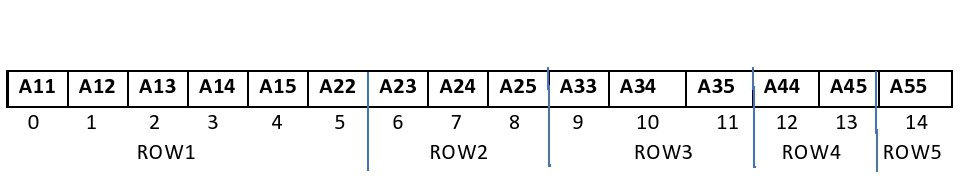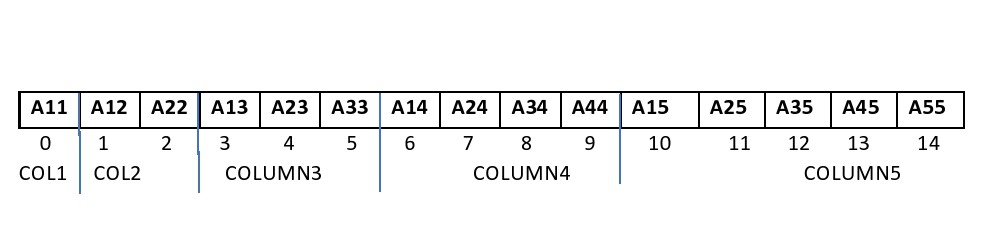Related Articles

# Convert given lower triangular Matrix to 1D array

• Difficulty Level : Medium
• Last Updated : 15 Feb, 2021

Given a lower triangular matrix M[][] of dimension N * N, the task is to convert it into a one-dimensional array by storing only non-zero elements.

Examples:

Input: M[][] = {{1, 0, 0, 0}, {2, 3, 0, 0}, {4, 5, 6, 0}, {7, 8, 9, 10}}
Output:
Row-wise: {1, 2, 3, 4, 5, 6, 7, 8, 9, 10}
Column-wise: {1, 2, 4, 7, 3, 5, 8, 6, 9, 10}
Explanation: All the non-zero elements of the matrix are {1, 2, 3, 4, 5, 6, 7, 8, 9, 10}.
Arranging these elements in row-wise manner in a 1D array generates the sequence {1, 2, 3, 4, 5, 6, 7, 8, 9, 10}.
Arranging these elements in column-wise manner in a 1D array generates the sequence {1, 2, 4, 7, 3, 5, 8, 6, 9, 10}.

Input: M[][] = {{1, 0, 0, }, {2, 3, 0}, {4, 5, 6}}
Output:
Row-wise: {1, 2, 3, 4, 5, 6}
Column-wise: {1, 2, 4, 3, 5, 6}

Approach:To convert a 2-dimensional matrix to a 1-dimensional array following two methods are used:

• In this method, adjacent elements of a row are placed next to each other in the array.• The following formula is used to find out the respective positions of the non-zero elements of the lower triangular matrix in the 1-dimensional array.

Index of matrix element at position (i, j) = ((i * (i – 1))/2 + j – 1)
where 1 ≤ i, j ≤ N and i ≥ j

• In this method, consecutive elements of a column are placed adjacently in the array.• The following formula is used to find out the respective positions of the non-zero elements of the lower triangular matrix in the 1-dimensional array.

Index of matrix element at position (i, j) = (N * (j – 1) – ((j – 2) * (j – 1))/2) + (i – j)
where 1 ≤ i, j ≤ N and i ≥ j

•

Follow the steps below to solve the problem:

• Initialize an array, say A[], to store the non-zero elements of the matrix.
• Traverse the matrix M[][] and find the index of non-zero elements of the matrix in the array A[] using the formula for row-major mapping and insert each non-zero element in the array A[].
• After completing the above step, print the array A[] for row-major mapping.
• Again, traverse the matrix M[][] and find the index of non-zero elements of the matrix in the array A[] using the formula for column-major mapping and insert each non-zero element in the array A[].
• After completing the above steps, print the array A[] for column-major mapping.

Below is the implementation of the above approach:

## C++

 `// C++ program for the above approach``#include ``using` `namespace` `std;` `// Class of Lower Triangular Matrix``class` `LTMatrix {` `private``:``    ``// Size of Matrix``    ``int` `n;` `    ``// Pointer``    ``int``* A;` `    ``// Stores the count of non-zero``    ``// elements``    ``int` `tot;` `public``:``    ``// Constructor``    ``LTMatrix(``int` `N)``    ``{``        ``this``->n = N;``        ``tot = N * (N + 1) / 2;``        ``A = ``new` `int``[N * (N + 1) / 2];``    ``}` `    ``// Destructor``    ``~LTMatrix() { ``delete``[] A; }` `    ``// Function to display array``    ``void` `Display(``bool` `row = ``true``);` `    ``// Function to generate array``    ``// in Row - Major order``    ``void` `setRowMajor(``int` `i, ``int` `j, ``int` `x);` `    ``// Function to generate array``    ``// in Column - Major order``    ``void` `setColMajor(``int` `i, ``int` `j, ``int` `x);` `    ``// Function to find size of array``    ``int` `getN() { ``return` `n; }``};` `// Function to generate array from``// given matrix by storing elements``// in column major order``void` `LTMatrix::setColMajor(``    ``int` `i, ``int` `j, ``int` `x)``{``    ``if` `(i >= j) {` `        ``int` `index``            ``= (n * (j - 1)``               ``- (((j - 2)``                   ``* (j - 1))``                  ``/ 2))``              ``+ (i - j);` `        ``A[index] = x;``    ``}``}` `// Function to generate array from``// given matrix by storing elements``// in row major order``void` `LTMatrix::setRowMajor(``    ``int` `i, ``int` `j, ``int` `x)``{``    ``if` `(i >= j) {``        ``int` `index = (i * (i - 1)) / 2``                    ``+ j - 1;``        ``A[index] = x;``    ``}``}` `// Function to display array elements``void` `LTMatrix::Display(``bool` `row)``{``    ``for` `(``int` `i = 0; i < tot; i++) {``        ``cout << A[i] << ``" "``;``    ``}``    ``cout << endl;``}` `// Function to generate and display``// array in Row-Major Order``void` `displayRowMajor(``int` `N)``{``    ``LTMatrix rm(N);` `    ``// Generate the array in the``    ``// row-major form``    ``rm.setRowMajor(1, 1, 1);``    ``rm.setRowMajor(2, 1, 2);``    ``rm.setRowMajor(2, 2, 3);``    ``rm.setRowMajor(3, 1, 4);``    ``rm.setRowMajor(3, 2, 5);``    ``rm.setRowMajor(3, 3, 6);``    ``rm.setRowMajor(4, 1, 7);``    ``rm.setRowMajor(4, 2, 8);``    ``rm.setRowMajor(4, 3, 9);``    ``rm.setRowMajor(4, 4, 10);` `    ``// Display array elements``    ``// in row-major order``    ``cout << ``"Row-Wise:\n"``;` `    ``rm.Display();``}` `// Function to generate and display``// array in Column-Major Order``void` `displayColMajor(``int` `N)``{``    ``LTMatrix cm(N);` `    ``// Generate array in``    ``// column-major form``    ``cm.setColMajor(1, 1, 1);``    ``cm.setColMajor(2, 1, 2);``    ``cm.setColMajor(2, 2, 3);``    ``cm.setColMajor(3, 1, 4);``    ``cm.setColMajor(3, 2, 5);``    ``cm.setColMajor(3, 3, 6);``    ``cm.setColMajor(4, 1, 7);``    ``cm.setColMajor(4, 2, 8);``    ``cm.setColMajor(4, 3, 9);``    ``cm.setColMajor(4, 4, 10);` `    ``// Display array elements``    ``// in column-major form``    ``cout << ``"Column-Wise:\n"``;``    ``cm.Display(``false``);``}` `// Driver Code``int` `main()``{``    ``// Size of row or column``    ``// of square matrix``    ``int` `N = 4;` `    ``// Function Call for row major``    ``// mapping``    ``displayRowMajor(N);` `    ``// Function Call for column``    ``// major mapping``    ``displayColMajor(N);` `    ``return` `0;``}`

## Java

 `// Java program for the above approach``import` `java.io.*;``class` `GFG``{` `    ``// Class of Lower Triangular Matrix``    ``static` `class` `LTMatrix``    ``{` `        ``// Size of Matrix``        ``static` `int` `n;` `        ``// Pointer``        ``static` `int` `A[];` `        ``// Stores the count of non-zero``        ``// elements``        ``static` `int` `tot;` `        ``// Constructor``        ``LTMatrix(``int` `N)``        ``{``            ``this``.n = N;``            ``tot = N * (N + ``1``) / ``2``;``            ``A = ``new` `int``[N * (N + ``1``) / ``2``];``        ``}` `        ``// Function to display array elements``        ``static` `void` `Display(``boolean` `row)``        ``{``            ``for` `(``int` `i = ``0``; i < tot; i++)``            ``{``                ``System.out.print(A[i] + ``" "``);``            ``}``            ``System.out.println();``        ``}` `        ``// Function to generate array from``        ``// given matrix by storing elements``        ``// in row major order``        ``static` `void` `setRowMajor(``int` `i, ``int` `j, ``int` `x)``        ``{``            ``if` `(i >= j) {``                ``int` `index = (i * (i - ``1``)) / ``2` `+ j - ``1``;``                ``A[index] = x;``            ``}``        ``}` `        ``// Function to generate array from``        ``// given matrix by storing elements``        ``// in column major order``        ``static` `void` `setColMajor(``int` `i, ``int` `j, ``int` `x)``        ``{``            ``if` `(i >= j) {` `                ``int` `index = (n * (j - ``1``)``                             ``- (((j - ``2``) * (j - ``1``)) / ``2``))``                            ``+ (i - j);``                ``A[index] = x;``            ``}``        ``}` `        ``// Function to find size of array``        ``static` `int` `getN() { ``return` `n; }``    ``}` `    ``// Function to generate and display``    ``// array in Row-Major Order``    ``static` `void` `displayRowMajor(``int` `N)``    ``{``        ``LTMatrix rm = ``new` `LTMatrix(N);` `        ``// Generate the array in the``        ``// row-major form``        ``rm.setRowMajor(``1``, ``1``, ``1``);``        ``rm.setRowMajor(``2``, ``1``, ``2``);``        ``rm.setRowMajor(``2``, ``2``, ``3``);``        ``rm.setRowMajor(``3``, ``1``, ``4``);``        ``rm.setRowMajor(``3``, ``2``, ``5``);``        ``rm.setRowMajor(``3``, ``3``, ``6``);``        ``rm.setRowMajor(``4``, ``1``, ``7``);``        ``rm.setRowMajor(``4``, ``2``, ``8``);``        ``rm.setRowMajor(``4``, ``3``, ``9``);``        ``rm.setRowMajor(``4``, ``4``, ``10``);` `        ``// Display array elements``        ``// in row-major order``        ``System.out.println(``"Row-Wise:"``);``        ``rm.Display(``false``);``    ``}` `    ``// Function to generate and display``    ``// array in Column-Major Order``    ``static` `void` `displayColMajor(``int` `N)``    ``{``        ``LTMatrix cm = ``new` `LTMatrix(N);` `        ``// Generate array in``        ``// column-major form``        ``cm.setColMajor(``1``, ``1``, ``1``);``        ``cm.setColMajor(``2``, ``1``, ``2``);``        ``cm.setColMajor(``2``, ``2``, ``3``);``        ``cm.setColMajor(``3``, ``1``, ``4``);``        ``cm.setColMajor(``3``, ``2``, ``5``);``        ``cm.setColMajor(``3``, ``3``, ``6``);``        ``cm.setColMajor(``4``, ``1``, ``7``);``        ``cm.setColMajor(``4``, ``2``, ``8``);``        ``cm.setColMajor(``4``, ``3``, ``9``);``        ``cm.setColMajor(``4``, ``4``, ``10``);` `        ``// Display array elements``        ``// in column-major form``        ``System.out.println(``"Column-Wise:"``);``        ``cm.Display(``false``);``    ``}` `    ``// Driver Code``    ``public` `static` `void` `main(String[] args)``    ``{``      ` `        ``// Size of row or column``        ``// of square matrix``        ``int` `N = ``4``;` `        ``// Function Call for row major``        ``// mapping``        ``displayRowMajor(N);` `        ``// Function Call for column``        ``// major mapping``        ``displayColMajor(N);``    ``}``}` `// This code is contributed by Dharanendra L V.`
Output:
```Row-Wise:
1 2 3 4 5 6 7 8 9 10
Column-Wise:
1 2 4 7 3 5 8 6 9 10```

Time Complexity: O(N2)
Auxiliary Space: O(N2)

Attention reader! Don’t stop learning now. Get hold of all the important DSA concepts with the DSA Self Paced Course at a student-friendly price and become industry ready.  To complete your preparation from learning a language to DS Algo and many more,  please refer Complete Interview Preparation Course.

In case you wish to attend live classes with experts, please refer DSA Live Classes for Working Professionals and Competitive Programming Live for Students.

My Personal Notes arrow_drop_up